﻿ Nonlinear Analysis of the Enclosure of the Pulley

### Nonlinear Analysis of the Enclosure of the Pulley

Pavol Lengvarský, Jozef Bocko, Martin Hagara

American Journal of Mechanical Engineering

## Nonlinear Analysis of the Enclosure of the Pulley

Pavol Lengvarský1,, Jozef Bocko1, Martin Hagara1

1Department of Applied Mechanics and Mechanical Engineering, Faculty of Mechanical Engineering, Technical University of Košice, 042 00 Košice, Slovak Republic

### Abstract

Nonlinear analysis of the enclosure of the pulley is described in this paper. Three different materials were used for the enclosure. Polyethylene, aluminium alloy and titanium alloy were used for model of the enclosure. The enclosure was pressed on the pulley and this frictional contact with friction coefficient was used in nonlinear finite element analysis. The maximal von Misses stress of the enclosure was higher than tensile strength of the materials used for the enclosure so three different variants were proposed. The maximal stress level of the three new variants was smaller than the yield point of the materials.

• Pavol Lengvarský, Jozef Bocko, Martin Hagara. Nonlinear Analysis of the Enclosure of the Pulley. American Journal of Mechanical Engineering. Vol. 3, No. 6, 2015, pp 220-224. http://pubs.sciepub.com/ajme/3/6/13
• Lengvarský, Pavol, Jozef Bocko, and Martin Hagara. "Nonlinear Analysis of the Enclosure of the Pulley." American Journal of Mechanical Engineering 3.6 (2015): 220-224.
• Lengvarský, P. , Bocko, J. , & Hagara, M. (2015). Nonlinear Analysis of the Enclosure of the Pulley. American Journal of Mechanical Engineering, 3(6), 220-224.
• Lengvarský, Pavol, Jozef Bocko, and Martin Hagara. "Nonlinear Analysis of the Enclosure of the Pulley." American Journal of Mechanical Engineering 3, no. 6 (2015): 220-224.

 Import into BibTeX Import into EndNote Import into RefMan Import into RefWorks

### 1. Introduction

The pulleys are parts of many engineering devices that are used in common practice. The pulleys work in different environment and accordingly they are made of different materials. The divided pulley consisting two parts is designed in our case and these parts are pressed together. The first part is pulley and the second is the enclosure. The enclosure from three different materials for the pulley was proposed by the authors. The enclosures were placed on the pulley (Figure 1). The maximal diameter of the pulley is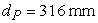and the maximal width of the pulley is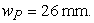The groove on the enclosure on of the pulley has dimensions: diameter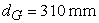and width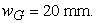Download asVeiw figureFigures index
Figure 1. The pulley

### 2. Basic of Nonlinear Theory

The computation of a stress and a deformation of structures in a lot of the engineering problems can be solved with the linear approximation. In linear simulations by the finite element method we use linear algebraic equations in form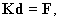(1)

where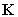is global stiffness matrix,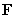is resultant vector of load forces and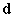is displacement vector of the whole structure .

If the relation between the load and the response is nonlinear, the structure is called nonlinear and the simulations are called the nonlinear simulations. All structures are nonlinear from different reasons, but we often neglect these nonlinearities and we use linear solutions. In some cases we cannot neglect nonlinearities and the nonlinear solutions are needed [1, 2].

We know tree basic types of nonlinearities: large deformation, change connectivity and nonlinear stress-strain relations. Nonlinearity due to large deformation is called geometry nonlinearity. Nonlinearity due to the connectivity change is called topology nonlinearity, which include failure of structural members and materials and change of contact status. Nonlinearity due to the nonlinear stress-strain relations is called material nonlinearity [1, 2, 3, 4].

In nonlinear simulation, the relation among nodal force and nodal displacement is nonlinear as shown in Figure 2 [1, 3].

In nonlinear cases matrixin the equation (1) is no longer a constant matrix, it changes with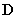and we can this equation write as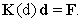(2)Download asVeiw figureFigures index
Figure 2. Linear and nonlinear functions

The loading history in nonlinear finite element analysis is defined by time or small substeps. The loading history is divided to small time increments [1, 2].

Nonlinear equation can be computed for instance by Newton-Raphson method, modified Newton-Raphson method, line search, force convergence and displacement convergence [1, 2, 3, 4].

### 3. Nonlinear Analysis

The pulley and the enclosure were proposed from two parts. These two parts will be joined by the hydraulic press. Due to fact that the enclosure is stretched and shifted during pressing, the nonlinear analyses for the enclosures made of three different materials were performed. The nonlinear analyses were performed by the finite element program ANSYS [5, 6, 7, 8, 9].

The material properties for the both parts of the structure are given in Table 1.

#### Table 1. Material properties

The basic dimensions of the pulley and the enclosure are shown in Figure 3. The inside diameter of the enclosure is smaller than the diameter of the groove in the pulley.Download asVeiw figureFigures index
Figure 3. Design of the pulley and the enclosure

The quarter model with symmetric boundary conditions (Figure 4) has been used for computations. The pulley was fixed (Figure 5) and on this pulley the enclosure was pressed. The frictional contact with frictional coefficients (steel-polyethylene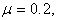steel-aluminium alloy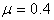and steel-titanium alloy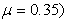was set .Download asVeiw figureFigures index
Figure 4. The finite element meshDownload asVeiw figureFigures index
Figure 5. The surfaces with applied boundary conditions on the quarter model

The mesh of finite elements (Figure 6) was generated automatically, but the contact sizing option was used for creating fine mesh in contact area and for the better convergence. Approximately 400 000 nodes and 190 000 elements were generated for the quarter model of structures.Download asVeiw figureFigures index
Figure 6. The finite element mesh

The process of the pressing of the enclosure on the pulley in nonlinear finite element analysis is shown in Figure 7.Download asVeiw figureFigures index
Figure 7. The process of the pressing

The results of the maximal equivalent von Misses stress were computed by nonlinear analysis. The maximal equivalent stress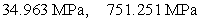and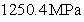was computed for the polyethylene, aluminium and titanium enclosure, respectively. The maximal stress is on the bottom part of the enclosure for all materials, the stress plots of the enclosures are shown in Figure 8 – Figure 10.Download asVeiw figureFigures index
Figure 8. Stress plot of the polyethylene enclosure on the pulleyDownload asVeiw figureFigures index
Figure 9. Stress plot of the aluminium enclosure on the pulley

These stresses in structures exceed the tensile strength of those materials so three new different variants of the pulley and the enclosure were proposed.Download asVeiw figureFigures index
Figure 10. Stress plot of the titanium enclosure on the pulley

The arc edges of the pulley were decreased in the first new variant as is shown in Figure 11.Download asVeiw figureFigures index
Figure 11. The first variant of both parts

The arc edges of the pulley were removed and the small edges on the enclosure were added as is shown in Figure 12.Download asVeiw figureFigures index
Figure 12. The second variant of both partsDownload asVeiw figureFigures index
Figure 13. The third variant of both parts

The third variant is almost the same as the second variant, but the groove is bigger so wider belt can be used (Figure 13).

The new nonlinear simulations with the same boundary, symmetric and contact conditions were performed for all modified variants.

The new computed results of the maximal von Misses stress for all variants are given in Table 2.

#### Table 2. Obtained results of maximal von Misses stress

Von Misses stresses of all new variants are smaller than yield points of materials. The maximal stress is on the bottom part for the all enclosures, the stress plots for all materials are shown in Figure 14 – Figure16. The maximal reaction forces for all variants are given in Table 3.Download asVeiw figureFigures index
Figure 14. Stress plot of the second variant for the polyethylene enclosure on the pulleyDownload asVeiw figureFigures index
Figure 15. Stress plot of the second variant for the aluminium enclosure on the pulleyDownload asVeiw figureFigures index
Figure 16. Stress plot of the second variant for the titanium enclosure on the pulley

### 4. Conclusion

The pressing of the enclosure made of three different materials on the pulley was performed by nonlinear finite element analysis. The whole pulley was modelled from two parts. Steel was used for the pulley and polyethylene, aluminium alloy and titanium alloy, respectively was used for the enclosure. The frictional contact with friction coefficients was set during simulation of pressing. The enclosure is pressed on the pulley 23 mm for the basic model and the first variant, 27.5 mm for the second and the third variant. The computed results for the whole structure were higher than tensile strength of the materials used for the enclosure and the pulley so three different modifications were proposed. Only small changes of design on the enclosure led to improvement of stress states on pulley. All computed results of these changed variants are given in table. Von Misses stresses of all new variants are smaller than yield points of materials.

### Acknowledgement

This article was created with support of VEGA grant project VEGA 1/1205/12 Numerical modelling of mechatronic systems and EU – OP “University science park Technicom for innovative applications with the support of sciential technologies” (ITMS: 26220220182).

### References

  Lee, H.H., Finite Element Simulations with ANSYS Workbench 14, SDC Publications, Mission, 2012.In article  Ivančo, V., Vodička, R., Numerické metódy mechaniky telies a vybrané aplikácie, SF TU, Košice, 2012.In article  Argyris, K.I., Hilout, S., Computational Methods in Nonlinear Analysis: Efficient Algorithms, Fixed Point Theory and Applications, World Scientific, Singapore, 2013.In article View Article  Kim, N.H., Introduction to Nonlinear Finite Element Analysis, Springer, NewYork, 2015.In article  Cyganiuk, J., “Pneumatic press for cold bending of metal elements,” Acta Mechanica Slovaca, 19 (2). 36-41. May.2015.In article  ZHOU, Z., et al., “Numerical simulation of warm compacted synchronous pulley,” Transactions of Nonferrous Metals Society of China, 16 (1). 65-70. Feb.2006.In article View Article  Evin, E., Nemeth, S., Vyrostek, M., “Evaluation of friction coefficient of stamping,” Acta Mechanica Slovaca, 18 (3-4). 20-27. 2014.In article  Yoon, S.Ch., Jeong, H.G., Lee, S., Kim, H.S., “Analysis of plastic deformation behavior during back pressure equal channel angular pressing by the finite element method,” Computational Materials Science, 77. 202-207. Sep.2013.In article View Article  Patil, B.V., Chakkingal, U., Kumar, T.S.P., “Effect of geometric parameters on strain, strain inhomogeneity and peak pressure in equal channel angular pressing,” Journal of Manufacturing Processes, 17. 88-97. Jan.2015.In article View Article  Kocich, R., “Macháčková, A., Andreyachshenko, V.A., “A study of plastic deformation behaviour of Ti alloy during equal channel angular pressing with partial back pressure,” Computational materials science, 101. 233-241. Apr.2015.In article View Article